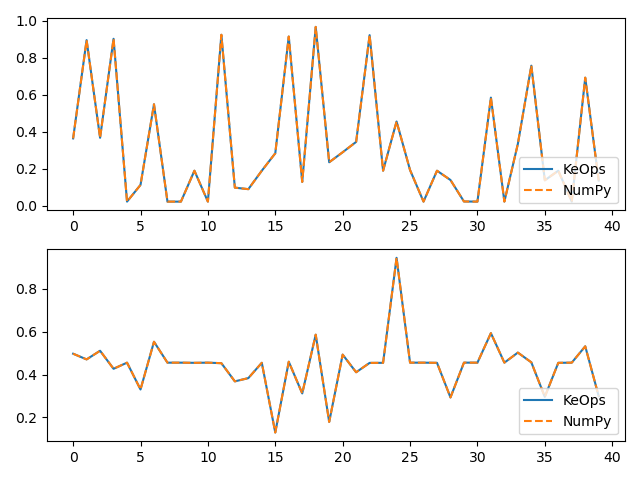SumSoftMaxWeight reduction¶

Using the numpy.Genred class, we show how to perform a computation specified through:

• Its inputs:

• $$x$$, an array of size $$M\times 3$$ made up of $$M$$ vectors in $$\mathbb R^3$$,

• $$y$$, an array of size $$N\times 3$$ made up of $$N$$ vectors in $$\mathbb R^3$$,

• $$b$$, an array of size $$N\times 2$$ made up of $$N$$ vectors in $$\mathbb R^2$$.

• Its output:

• $$c$$, an array of size $$M\times 2$$ made up of $$M$$ vectors in $$\mathbb R^2$$ such that

$c_i = \frac{\sum_j \exp(K(x_i,y_j))\,\cdot\,b_j }{\sum_j \exp(K(x_i,y_j))},$

with $$K(x_i,y_j) = \|x_i-y_j\|^2$$.

Setup¶

Standard imports:

import time
import numpy as np
from pykeops.numpy import Genred
import matplotlib.pyplot as plt

Define our dataset:

M = 5000  # Number of "i" points
N = 4000  # Number of "j" points
D = 3  # Dimension of the ambient space
Dv = 2  # Dimension of the vectors

x = 2 * np.random.randn(M, D)
y = 2 * np.random.randn(N, D)
b = np.random.rand(N, Dv)

KeOps kernel¶

Create a new generic routine using the numpy.Genred constructor:

formula = "SqDist(x,y)"
formula_weights = "b"
aliases = [
"x = Vi(" + str(D) + ")",  # First arg:  i-variable of size D
"y = Vj(" + str(D) + ")",  # Second arg: j-variable of size D
"b = Vj(" + str(Dv) + ")",
]  # Third arg:  j-variable of size Dv

softmax_op = Genred(
formula, aliases, reduction_op="SumSoftMaxWeight", axis=1, formula2=formula_weights
)

# Dummy first call to warmup the GPU and get accurate timings:
_ = softmax_op(x, y, b)

Use our new function on arbitrary Numpy arrays:

start = time.time()
c = softmax_op(x, y, b, backend="GPU_1D")
print("Timing (KeOps implementation): ", round(time.time() - start, 5), "s")

# compare with direct implementation
start = time.time()
cc = np.sum((x[:, None, :] - y[None, :, :]) ** 2, axis=2)
cc -= np.max(cc, axis=1)[:, None]  # Subtract the max to prevent numeric overflows
cc = np.exp(cc) @ b / np.sum(np.exp(cc), axis=1)[:, None]
print("Timing (Numpy implementation): ", round(time.time() - start, 5), "s")

print("Relative error : ", (np.linalg.norm(c - cc) / np.linalg.norm(c)).item())

# Plot the results next to each other:
for i in range(Dv):
plt.subplot(Dv, 1, i + 1)
plt.plot(c[:40, i], "-", label="KeOps")
plt.plot(cc[:40, i], "--", label="NumPy")
plt.legend(loc="lower right")
plt.tight_layout()
plt.show()Out:

Timing (KeOps implementation):  0.01096 s
Timing (Numpy implementation):  2.02406 s
Relative error :  7.178561569121771e-16

Total running time of the script: ( 0 minutes 2.468 seconds)

Gallery generated by Sphinx-Gallery